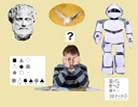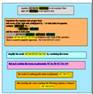QJF-RCCALQJF-RCCAL   EDUCATIONAL SYSTEM “LUMEN”EDUCATIONAL SYSTEM “LUMEN”Presented here is an example of one session works with the system “Lumen”.  The arbitrary algebraic equation is given to “Lumen” to solve it but the system needs to find a method for solving the equation. The system explains in details every step of obtaining the solution. This way of explanation does not use the interactive way of solving, very disturbing by asking questions in the form of “insert a number”. It is assumed that, after training on many non-trivial examples of mathematical problems, a student will be able not only to understand and solve a problem but also to explain each step of solving the problem. The system can also generate algebraic tasks with the similar difficulty level or solve the algebraic tasks that are given by user. At this stage the system “Lumen” is subjected to the complex testing procedure and will be developed to solve and to explain problems from the different domains such as physics or chemistry. There is a big stress to enrich the ability of Lumen to solve the text tasks as well as to understand explanations given by a student.   The system “Lumen” is intended to be part of the explanatory module of SUS. In the first version this module was used for explanation of problems to the other machine or to the user (student). For this reason, in the contrast to the Paris Group which stresses the importance of solving of the tests, our proposal is focused on teaching/learning of the explanatory ability of solved problems. Our definition of understanding consists of two parts, the first is referring to solving problems and the second to explanation of the solving process and obtained results. In this context the ability to explain the process of solving problems appears to be the most important part of understanding of the problem. Leaving the detail discussion of issue of teaching/learning of explanatory abilities that will be presented elsewhere, it is important to point out to one important aspect of this topic. It is the matter of “polishing” of the acquired knowledge during teaching/learning process. In the example that is shown, it is easy to notice that complex problems can be decomposed into sub-problems that could be solved independently. Deep understanding of the solving process is the best guarantee for obtaining the proper solution. Deep understanding assumes the ability to explain each step of obtaining the solution without paying too much attention to obtained result. Even when the result can be wrong as the result of, for example, some computational mistake, the proper explanation of the solving process will be assessed as the top mark. In the professional practice this type of complex problems will be solved by application of the special software packages such as Mathematica. To conclude, learning the ability to understand the process of solving problems that culminates in acquiring the very good ability to explain the way of obtaining the solution can put into end so fast spreading testing madness.       Copyright the Queen Jadwiga Foundation Level 0 L1,  L2,  L3,  L4,  L5   Level 1   Level 2   Example - Interactive model